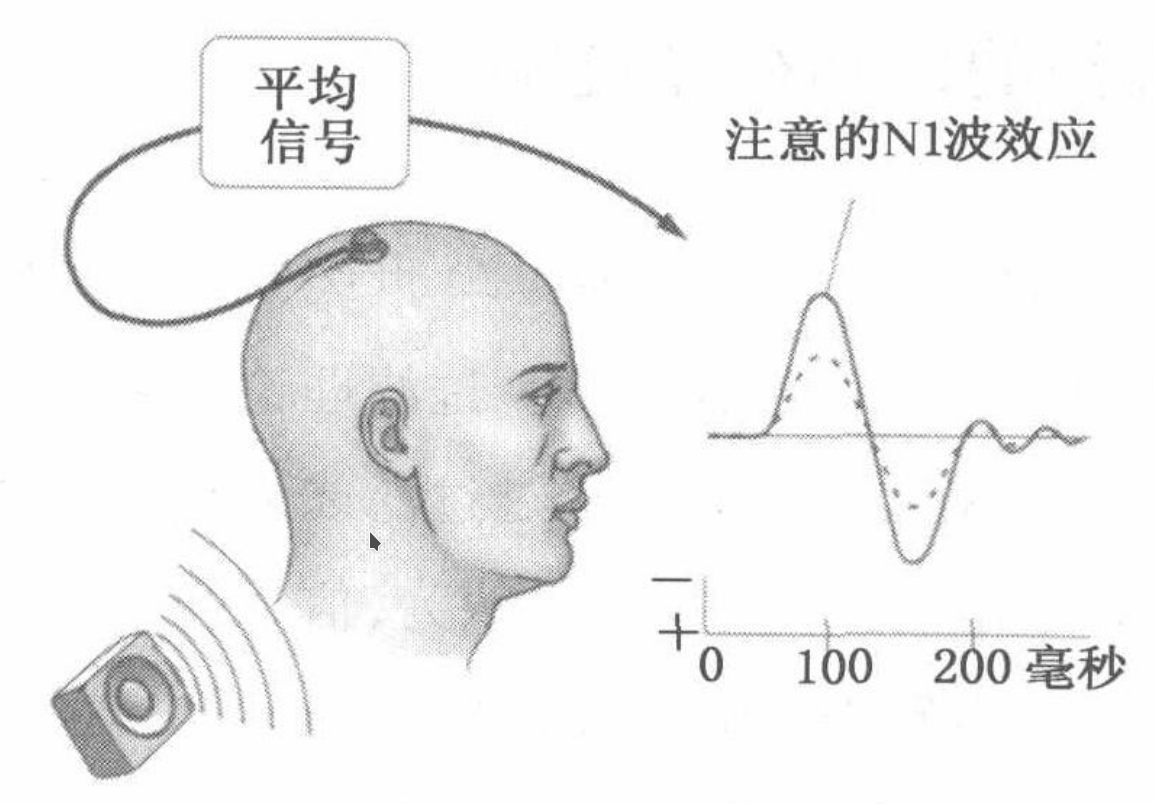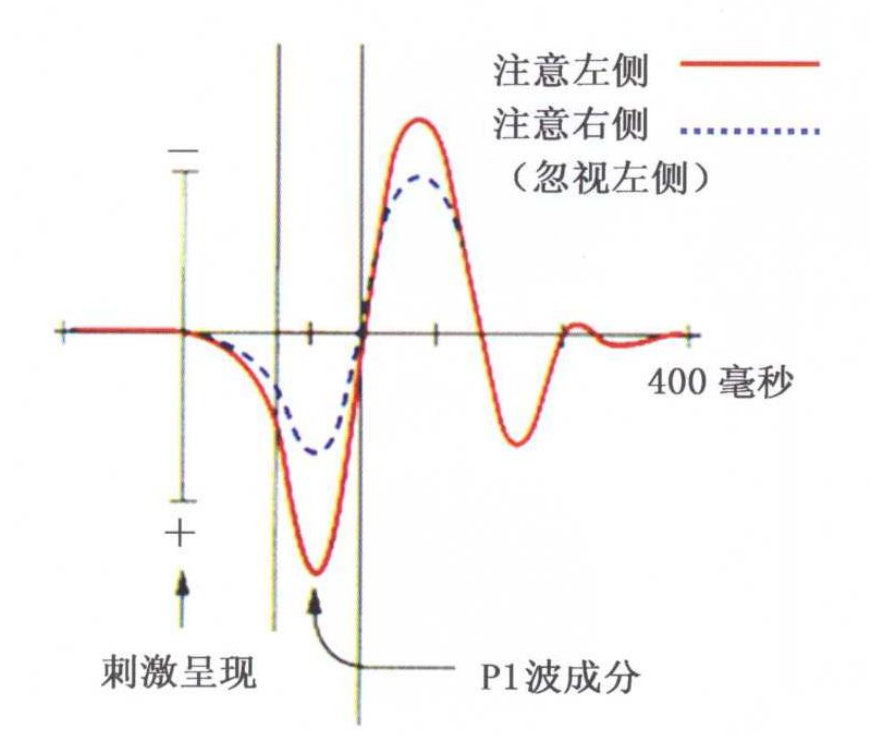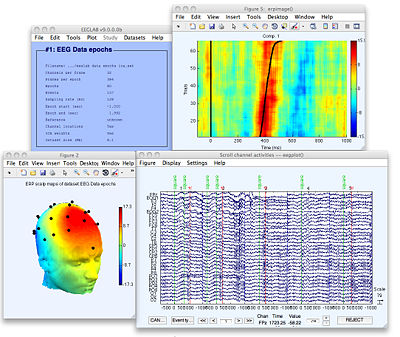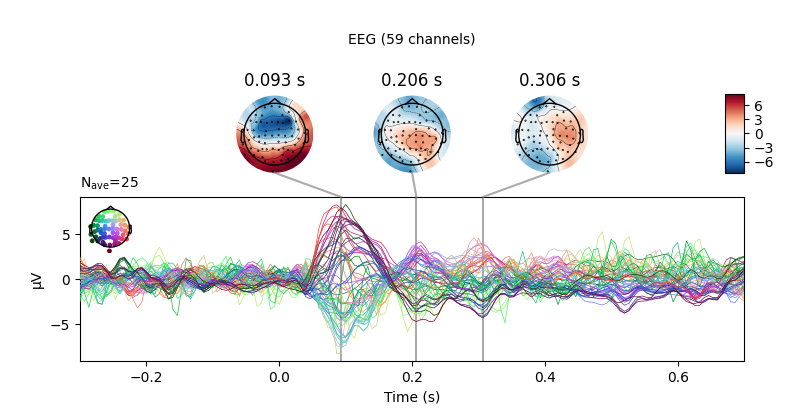# 事件相关电位¶

## 引言¶

ERPs可以通过脑电图(EEG)可靠地测量,脑电图是一种通过放置在头皮上的电极来测量随时间变化的大脑电活动的方法.脑电图反映了数千个同时进行的大脑过程.这意味着大脑对单一刺激或感兴趣的事件的反应通常在单个试验的脑电图记录中不可见.为了观察大脑对刺激的反应,实验者必须进行多次试验并将结果平均,使随机的大脑活动平均,相关的波形保持不变,这就是所谓的ERP.

An event-related potential (ERP) is the measured brain response that is the direct result of a specific sensory, cognitive, or motor event. More formally, it is any stereotyped electrophysiological response to a stimulus.

## 大脑产生的与事件相关的电压波动意味着什么?¶

ERP是神经信息处理的毫秒级记录,反应了大脑的感觉编码,抑制反应和更新工作记忆等特定的操作的神经活动.下面是笔者整理的几个例子,或许能够帮助读者理解事件相关电位的意义.

### 听觉注意¶

1973年,希利亚德设计了一个巧妙的实验,在一定程度上解释了听觉的注意机制.他们通过耳机在被试的两耳同时呈现不同音高的声音刺激流,在一种条件下要求被试注意呈现在左耳的刺激而忽略右耳,在另一种条件下则注意右耳的刺激而忽略左耳.这样,研究者就可以在被试只注意一侧耳刺激的条件下记录两个声音诱发的事件相关电位反应.结果发现,刺激注意与刺激忽略条件相比,听觉事件相关电位的振幅明显放大了.由于这个波是在刺激呈现后事件相关电位的第一个显著负波(N1波),而N1波成分是一种由感觉刺激诱发的脑波.所以,实验结果可能是感觉加工早期选择的证据.### 视觉注意¶## 事件相关电位如何提取和分析?¶

1. 在每次实验中,事件发生后段时间内,感兴趣的信号延迟和形状都不会变.

2. 根据中心极限定理,在实验的次数足够多时,过程中的干扰的均值服从均值为0,方差为$$\sigma ^2$$的正态分布

### 数学定义¶

$x(t,k) = s(t) + n(t,k).$

$\bar{x}(t) = \frac{1}{N}\sum^{N}_{k=1} x(t,k) = s(t) +\frac{1}{N}\sum^{N}_{k=1} n(t,k)$

$\operatorname{Var}[\bar{x}(t)]=\mathrm{E}\left[(\bar{x}(t)-\mathrm{E}[\bar{x}(t)])^{2}\right]=\frac{1}{N^{2}} \mathrm{E}\left[\left(\sum_{k=1}^{N} n(t, k)\right)^{2}\right]=\frac{1}{N^{2}} \sum_{k=1}^{N} \mathrm{E}\left[n(t, k)^{2}\right]=\frac{\sigma^{2}}{N}$

## 如何使用程序来分析ERP¶

### eeglab¶

eeglab是研究者们最常用的脑电分析工具### mne¶

mne是一个用于分析EEG,MEG,ECoG和NIRS等神经生理学数据的python库,相对于eeglab来说更加灵活.

epochs.plot_joint()